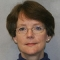Suppose that you want to know the value of a character variable that has the highest frequency count or even the top three highest values. To determine that value, you need to create an output data set and sort the data by the descending Count or _FREQ_ variable. Then you need to print the top n observations using the OBS= option, based on the number of values that you want to see. You can do this easily using any of a variety of procedures that calculate a frequency count (for example, the FREQ Procedure or the MEANS Procedure).

This blog provides two detailed examples: one calculates the top n values for a single variable and one calculates the top n values for all character variables in a data set.

### Print the top n observations of a single variable

The following example prints the three values of the Make variable in the Sashelp.Cars data set that have the highest frequency count. By default, PROC FREQ prints a variable called Count in the output data set. The output data set is sorted by this variable in descending order, and the number of observations that you want to keep is printed by using the OBS= data set option.

```proc freq data=sashelp.cars noprint; tables make / out=counts(drop=percent); run;   proc sort data=counts; by descending count; run;   proc print data=counts(obs=3); run;```

### Print the top n observations of all character variables in a data set

Suppose that you want to know the top three values for all the character variables in a data set. The process shown in the previous section is not efficient when you have many variables. Suppose you also want to store this information in a data set. You can use macro logic to handle both tasks. The following code uses PROC FREQ to create an output data set for each variable. Further manipulation is done in a DATA step so that all the data sets can be combined. A detailed explanation follows the example code:

```%macro top_frequency(lib=,dsn=);   /* count character variables in the data set */ proc sql noprint; select name into :charlist separated by ' ' from dictionary.columns where libname=%upcase("&lib") and memname=%upcase("&dsn")and type='char'; quit;   %put &charlist; %let cnt=%sysfunc(countw(&charlist,%str( ))); %put &cnt;   %do i=1 %to &cnt;   /* Loop through each character variable in */ /* FREQ and create a separate output */ /* data set. */ proc freq data=&lib..&dsn noprint; tables %scan(&charlist,&i) / missing out=out&i(drop=percent rename=(%scan(&charlist,&i)=value)); run;   data out&i; length varname value \$100; set out&i; varname="%scan(&charlist,&i)"; run;   proc sort data=out&i; by varname descending count; run;   %end;   data combine; set %do i=1 %to &cnt; out&i(obs=3) /* Keeps top 3 for each variable. */ %end;; run;   proc print data=combine; run;   %mend top_frequency;   options mprint mlogic symbolgen; %top_frequency(lib=SASHELP,dsn=CARS);```

I begin my macro definition with two keyword parameters that enable me to substitute the desired library and data set name in my macro invocation:

`%macro top_frequency(lib=,dsn=);`

The SQL procedure step selects all the character variables in the data set and stores them in a space-delimited macro variable called &CHARLIST. Another macro variable called &CNT counts how many words (or, variable names) are in this list.

```proc sql noprint; select name into :charlist separated by ' ' from dictionary.columns where libname=%upcase("&lib") and memname=%upcase("&dsn") and type='char'; quit;   %put &charlist; %let cnt=%sysfunc(countw(&charlist,%str( ))); %put &cnt;```

The %DO loop iterates through each variable in the list and generates output data from PROC FREQ by using the OUT= option. The output data set contains two variables: the variable from the TABLES request with the unique values of that variable and the Count variable with the frequency counts. The variable name is renamed to Value so that all the data sets can be combined in a later step. In a subsequent DATA step, a new variable, called Varname, is created that contains the variable name as a character string. Finally, the data set is sorted by the descending frequency count.

```%do i=1 %to &cnt;   /* Loop through each character variable in PROC FREQ */ /* and create a separate output data set. */ proc freq data=&lib..&dsn noprint; tables %scan(&charlist,&i) / missing out=out&i(drop=percent rename=(%scan(&charlist,&i)=value)); run;   data out&i; length varname value \$100; set out&i; varname="%scan(&charlist,&i)"; run;   proc sort data=out&i; by varname descending count; run;   %end;```

The final DATA step combines all the data sets into one using another macro %DO loop in the SET statement. The %END statement requires two semicolons: one ends the SET statement and one ends the %END statement. Three observations of each data set are printed by using the OBS= option.

```data combine; set %do i=1 %to &cnt; out&i(obs=3) /* Keeps top 3 for each variable. */ %end;; run;```

Knowing your data is essential in any programming application. The ability to quickly view the top values of any or all variables in a data set can be useful for identifying top sales, targeting specific demographic segments, trying to understand the prevalence of certain illnesses or diseases, and so on. As explained in this blog, a variety of Base SAS procedures along with the SAS macro facility make it easy to accomplish such tasks.

These resources show different ways to create "top N" reports in SAS:

SharePrincipal Technical Support Analyst

Kathryn McLawhorn has worked in Technical Support at SAS since 1997. She started as a consultant in the Base Procedures and ODS group, and she is currently a consultant in the CAS/Open Source Languages and SAS Programming group. She primarily supports report writing, ODS, and Base summary procedures. Kathryn has her SAS Certification in both Base Programming for SAS 9 and Advanced Programming for SAS 9.

1.Hi. You could eliminate the sort with a PROC FREQ option ...

proc freq data=sashelp.cars noprint order=freq;
tables make / out=counts (drop=percent);
run;

•Kathryn McLawhorn on

Thanks for pointing that out.
Kathryn

This site uses Akismet to reduce spam. Learn how your comment data is processed.